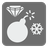Inertia
Elementary+
English RU

This mission is an adaptation of the "Inertia" game (from Simon Tatham's Portable Puzzle Collection). If you are lost or just want to play, the game is available here.

You're on a rectangular mined icefield: when you go in one direction, you slide on the ice until you're stopped by a rock, the end of the icefield, or some rough spot. But you're here for a good reason, there are gems on the icefield: you have to find any route to collect them all, avoiding the mines.

The icefield will be represented by a tuple of strings. Gems will be represented by '\$', rocks by 'X', mines by '*', ice by ' ' and rough spots by '.'.

You can go in all eight directions: northwest 'NW', north 'N', northeast 'NE', west 'W', east 'E', southwest 'SW', south 'S', southeast 'SE'.

```Dead route (you're stuck): ['SE', 'E', 'SE']

Possible route: ['SE', 'E', 'W', 'NW', 'N',
'S', 'SW', 'S', 'S', 'NE',
'E', 'SE', 'E', 'N', 'SW',
'NW', 'NW', 'SE', 'NE',
'NW', 'E', 'NE', 'N']

grid = (' .\$*.X*X*\$',        start = (0, 1)
'.X\$** X X\$',
' *.\$ *X..X',
'*.*. \$XX\$X',
'  .  X.* .',
' .*X.X\$*\$X',
'.\$\$  .*.X\$',
'*\$*.*X\$\$\$ ')
```

Input: A tuple of strings and a tuple of two ints.

Output: An iterable of strings.

Example:

```list(checkio((' .\$*.X*X*\$',
'.X\$** X X\$',
' *.\$ *X..X',
'*.*. \$XX\$X',
'  .  X.* .',
' .*X.X\$*\$X',
'.\$\$  .*.X\$',
'*\$*.*X\$\$\$ '), (0, 1))) = ['SE', 'E', 'W', 'NW', 'N',
'S', 'SW', 'S', 'S', 'NE',
'E', 'SE', 'E', 'N', 'SW',
'NW', 'NW', 'SE', 'NE',
'NW', 'E', 'NE', 'N']
```

To play the puzzles / tests yourself: 1 2 3 4 5 6 7 8 9 10 11 12 13 14 15 16 17

Preconditions:

• You can collect all gems in any possible order (otherwise it would be far more difficult).
• Given puzzles are solvable.
• 5 ≤ len(grid) ≤ 50 and 5 ≤ len(grid) ≤ 50.
• all(len(row) == len(grid) for row in grid).